# Linear Probability Forecasting

This generalization of online linear regression framework describes a way of predicting outcomes from the probability simplex. If there is a set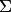with finite number of elements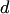, we say that each outcome belongs to the space of all probability measures over,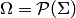.

In this setting, each outcome hascomponents. A natural algorithm to predict such an outcome is the coordinate-wise application of the Aggregating Algorithm Regression. The equivalent algorithm is derived if all the experts are mixed directly using the Aggregating Algorithm. However, if the outcome belongs to the simplex, simple linear experts are not very efficient: they give predictions lying in the whole space. In this paper, more efficient linear experts are considered. Their predictions lie in the hyperplane of the simplex.

Learner competes with all linear functions (experts)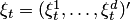of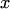: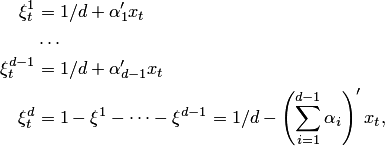where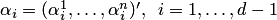. In the model~\eqref{eq:experts1} the prediction for the last component of the outcome is calculated from the predictions for other components. Denote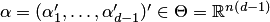. Then any expert can be represented as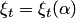.

Two algorithms are applied. The first just uses component-wise prediction of the AAR, and then projects it to the hyperplane. It is proven that this algorithm competes with the necessary experts. It achieves the following bound.

Theorem. If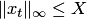for all, then for any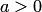, every positive integer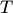, every sequence of outcomes of the length, and any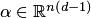, the loss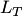of the component-wise algorithm satisfies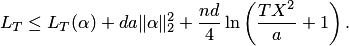The second algorithms mixes all the experts directly, and is called mAAR (multidimensional AAR). It achieves the following bound.

Theorem. Iffor all, then for any, every positive integer, every sequence of outcomes of the length, and any, the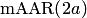satisfies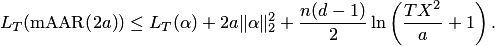The second upper bound~ has worse constant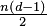in front of the logarithm than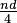of the first bound if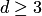. In this case the second bound is worse asymptotically inthan the first one, but it is better in the beginning, especially when the norm of the best expert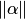is large. This can happen in the important case when the dimension of the input vectors is larger than the size of the prediction set: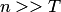.

Experiments showed that on 5 databases the online performance is better than batch logistic regression (trained once), but worse than the online logistic regression (retrained every step). At the same time, the speed is faster than the online logistic regression.

### Bibliography

• F. Zhdanov and Y. Kalnishkan. Linear Probability Forecasting, to appear in AIAI 2010 proceedings.